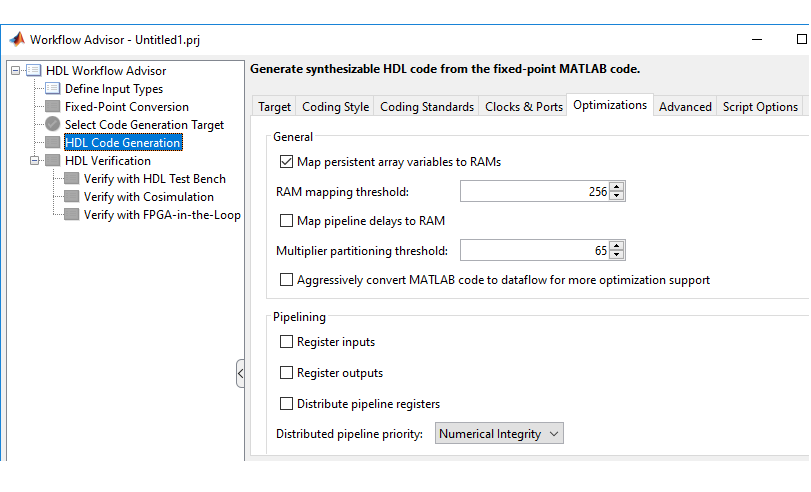## Map Persistent Arrays and dsp.Delay to RAM

### How To Enable RAM Mapping

1. In the HDL Workflow Advisor, select MATLAB to HDL Workflow > Code Generation > Optimizations tab.

2. Select the Map persistent array variables to RAMs option.

3. Set the RAM mapping threshold to the size (in bits) of the smallest persistent array, user-defined System object™ private property, or `dsp.Delay` that you want to map to RAM.### RAM Mapping Requirements for Persistent Arrays and System object Properties

The following table shows a summary of the RAM mapping behavior for persistent arrays and private properties of a user-defined System object.

Map Persistent Array Variables to RAMs SettingMapping Behavior

on

Map to RAM. For restrictions, see RAM Mapping Restrictions.

off

Map to registers in the generated HDL code.

#### RAM Mapping Restrictions

When you enable RAM mapping, a persistent array or user-defined System object private property maps to a block RAM when all of the following conditions are true:

• Each read or write access is for a single element only. For example, submatrix access and array copies are not allowed.

• Persistent variables or user-defined System object private properties are initialized to 0 if they have a cyclic dependency. For example, if you have two persistent variables, A and B, you have a cyclic dependency if A depends on B, and B depends on A.

• If an access is within a conditional statement, the conditional statement uses only simple logic expressions (`&&`, `||`, `~`) or relational operators. For example, in the following code, `r1` does not map to RAM:

```if (mod(i,2) > 0) a = r1(u); else r1(i) = u; end ```

Rewrite complex conditions, such as conditions that call functions, by assigning them to temporary variables, and using the temporary variables in the conditional statement. For example, to map `r1` to RAM, rewrite the previous code as follows:

```temp = mod(i,2); if (temp > 0) a = r1(u); else r1(i) = u; end ```

• The persistent array or user-defined System object private property value depends on external inputs.

For example, in the following code, `bigarray` does not map to RAM because it does not depend on `u`:

```function z = foo(u) persistent cnt bigarray if isempty(cnt) cnt = fi(0,1,16,10,hdlfimath); bigarray = uint8(zeros(1024,1)); end z = u + cnt; idx = uint8(cnt); temp = bigarray(idx+1); cnt(:) = cnt + fi(1,1,16,0,hdlfimath) + temp; bigarray(idx+1) = idx; ```

• `RAMSize` is greater than or equal to the `RAMMappingThreshold` value. `RAMSize` is the product `NumElements * WordLength * Complexity`.

• `NumElements` is the number of elements in the array.

• `WordLength` is the number of bits that represent the data type of the array.

• `Complexity` is 2 for arrays with a complex base type; 1 otherwise.

If any of the above conditions is false, the persistent array or user-defined System object private property maps to a register in the HDL code.

### RAM Mapping Requirements for `dsp.Delay`System Objects

A summary of the mapping behavior for a `dsp.Delay` System object is in the following table.

Map Persistent Array Variables to RAMs OptionMapping Behavior

on

A `dsp.Delay` System object maps to a block RAM when all of the following conditions are true:

• `Length` property is greater than 4.

• `InitialConditions` property is 0.

• Delay input data type is one of the following:

• Real scalar with a non-floating-point data type.

• Complex scalar with real and imaginary parts that are non-floating-point.

• Vector where each element is either a non-floating-point real scalar or complex scalar.

• `RAMSize` is greater than or equal to the RAM Mapping Threshold value.

• `RAMSize` is the product `Length` * `InputWordLength`.

• `InputWordLength` is the number of bits that represent the input data type.

If any of the conditions are false, the `dsp.Delay` System object maps to registers in the HDL code.

off

A `dsp.Delay` System object maps to registers in the generated HDL code.